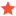Matlab API: How to get velocity and acceleration of robot continously?CaroSJunior MemberPosts: 1 Threads: 1 Joined: Nov 2019 Reputation: 0 11-08-2019, 09:04 AM I made a Simulink model with several Matlab function blocks to get data from the robot from RoboDK, edit that data and send it back to RoboDK.  One thing I need is the speed and acceleration of the robot TCP. I already wrote a function that calculates the speed from the time which went by when moving from one point to another.  Unfortunately, it seems like the block is executed to rare. When I visualize the velocity with a scope block I only get sort of bars and not a continous curve. Additionally the data seem to be implausible. Is there a way to get the velocity and acceleration continously and straight out of RoboDK? I got the tip to use the function InstructionListJoints, which should be in the class RobolinkItem. Unfortunately, it wasn't included there. Function code to calculate the speed: Code:```function [speedX, speedY, speedZ] = getSpeed(x, y, z) persistent x1 x2 y1 y2 z1 z2 t1 t2; if isempty(x1) % First function call -> Initialize parameters   x1=0; y1=0; z1=0; x2=x; y2=y; z2=z; t1=0; t2=0; speedX=0; speedY=0; speedZ=0; tic; % Start timer else % Shift the parameters x1=x2; y1=y2; z1=z2; t1=t2; % Get new values x2=x; y2=y1; z2=z1; t2=toc; % Get time since timerstart tic; % Start timer again % Calculate the velocity speedX = abs(x2-x1)/(t2-t1); speedY = abs(y2-y1)/(t2-t1); speedZ = abs(z2-z1)/(t2-t1); end end``` Find ReplyAlbertModeratorPosts: 1,053 Threads: 1 Joined: Apr 2018 Reputation: 50 11-11-2019, 07:11 PM You can use a function like this one to display the robot speed: https://github.com/RoboDK/RoboDK-API/blo...rJoints.py This is written in Python but you could do the same in Matlab. A more complete example is available here: C:/RoboDK/Macros/MonitorJoints.py You also need to derivate the position to calculate the speed. If you need the Cartesian speed (not the joint speed) you may want to use robot.Pose() to retrieve the 4x4 pose matrix instead of robot.Joints(). Also, keep in mind that if you run this on a parallel thread you'll need a new instance of Robolink(). Albert Find Reply